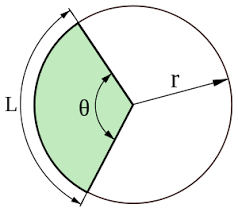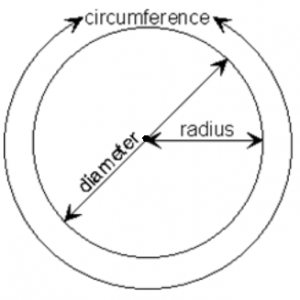## How to Calculate and Solve for the Angle, Radius and Length of an Arc of a Circle | The Calculator EncyclopediaThe image above represents the length of an arc of a circle.

To compute the length of an arc of a circle, two essential parameters are needed and this parameters are radius of the circle (r) and angle of the circle (α).

The formula for calculating the length of an arc of a circle:

L = απr / 180

Where;
L = Length of an arc of the circle
α = Angle of the circle
r = radius of the circle

Let’s solve an example:
Find the length of an arc of a circle when the angle of the circle is 90° and the radius of the circle is 20 cm.

This implies that;
α = Angle of the circle = 90°
r = Radius of the circle = 20 cm

L = απr / 180
L = 90 x 3.142 x 20 / 180
L = 5655.6 / 180
L = 31.42

Therefore, the length of an arc of the circle is 31.42 cm.

Calculating the Angle of a Circle using the Radius of the Circle and Length of an Arc of the Circle.

α = 180L / πr

Where;
L = Length of an arc of the circle
r = Radius of the circle

Let’s solve an example;
Find the angle of the circle when the length of an arc of the circle is 60° and a radius of 140 cm.

This implies that;
L = Length of an arc of the circle = 60°
r = Radius of the circle = 140 cm

α = 180L / πr
α = 180 x 60 / 3.142 x 140
α = 10800 / 439.88
a = 24.55

Therefore, the angle of the circle is 24.55°.

## How to Calculate and Solve for the Perimeter or Circumference, Radius and Diameter of a Circle | The Calculator EncyclopediaThe image above is a circle.

To compute the perimeter or circumference of a circle, one essential parameter is needed and this parameter is the radius of a circle (r). You can also use the diameter of a circle to compute the area of a circle (d).

The formula for calculating the perimeter or circumference of a circle is:

P = 2πr

Where:

P = Perimeter or Circumference of a circle
r = Radius of a circle

Let’s solve an example:
Find the perimeter or circumference of a circle where the radius of a circle is 8 cm.

This implies that;
r = Radius of a circle = 8 cm

P = 2πr
P = 2 x 3.142 x 8
P = 50.265

Therefore, the perimeter or circumference of a circle is 50.265 cm.

Calculating the Area of a Circle using the Diameter of a Circle.

The formula is P = πd

Where:

P = Perimeter or Circumference of a circle
d = Diameter of a circle

Let’s solve an example:
Find the perimeter or circumference of a circle where the diameter of a circle is 10 cm.

This implies that;
d = Diameter of a circle = 10 cm

P = πd
P = 3.142 x 10
P = 31.42

Therefore, the perimeter or circumference of a circle with diameter is 31.42 cm.

How to Calculate Radius of a Circle when Perimeter or Circumference of the Circle is Given

r = P /

where;

r = Radius of a circle
P = Perimeter or Circumference of a circle

Let’s solve an example:
Find the radius of a circle where the perimeter or circumference of the circle is 16 cm.

This implies that;
P = Perimeter or Circumference of the circle = 16 cm

r = P /
r = 16 / 6.284
r = 2.55

Therefore, the radius of the circle is 2.55 cm.

How to Calculate Diameter of a Circle when Perimeter or Circumference of the Circle is Given

d = P / π

where;

d = Diameter of a circle
P = Perimeter or Circumference of a circle

Let’s solve an example;
Find the diameter of a circle where the perimeter or circumference of the circle is 20 cm

This implies that;
P = Perimeter or Circumference of the circle = 20 cm

d = P / π
d = 20 / π
d = 6.365

Therefore, the diameter of the circle is 6.365 cm.